CBSE Class 10 Sample Paper for 2022 Boards - Maths Basic [Term 2]

Class 10
Solutions of Sample Papers for Class 10 Boards

## ii) Find the time taken by the boat to reach the light house if it is moving at the rate of 20 km per hour.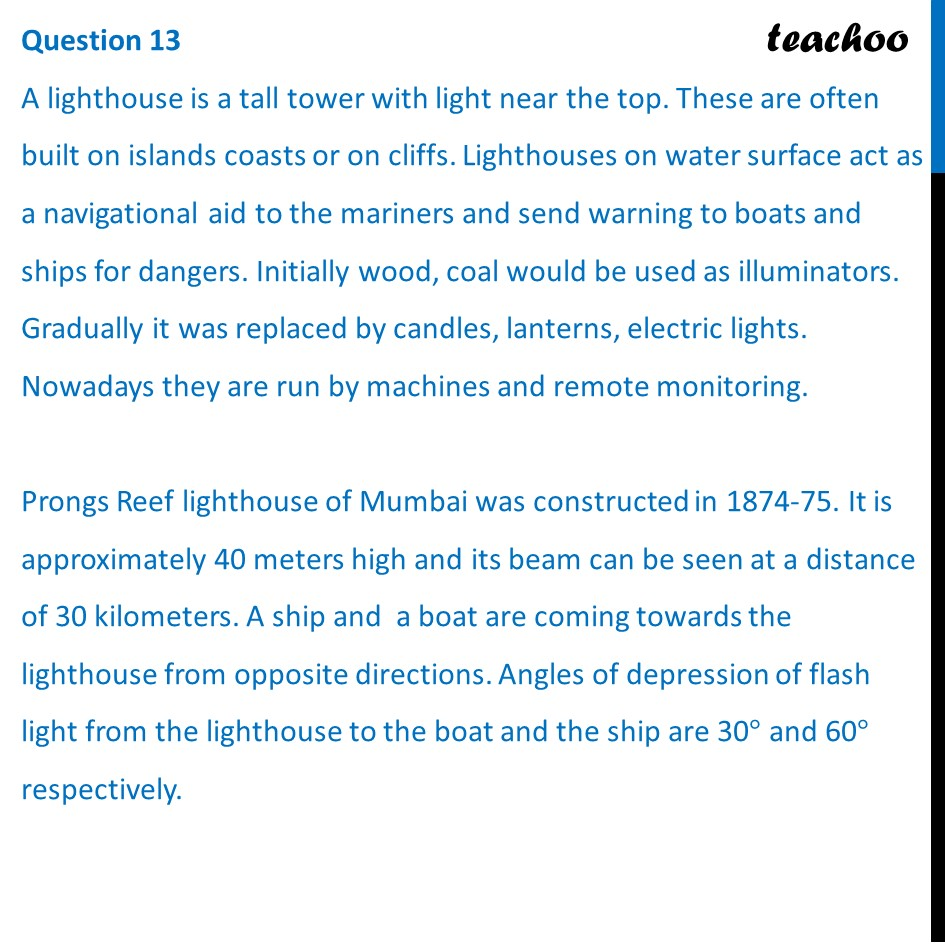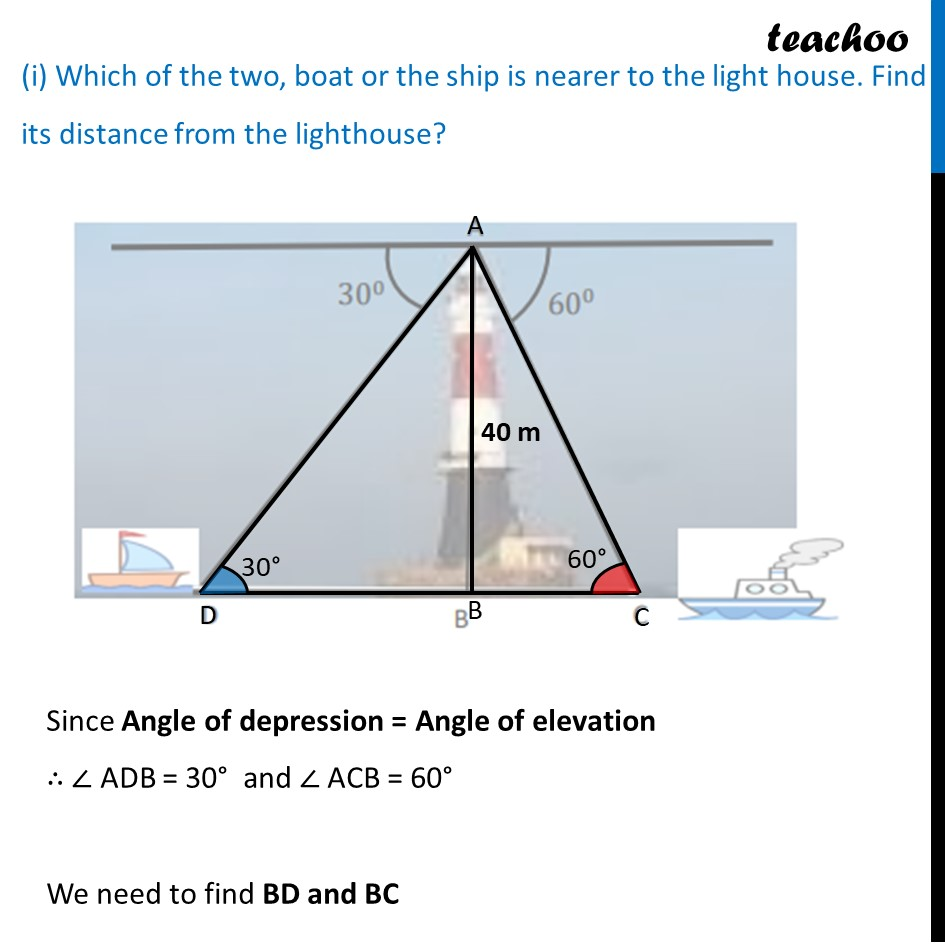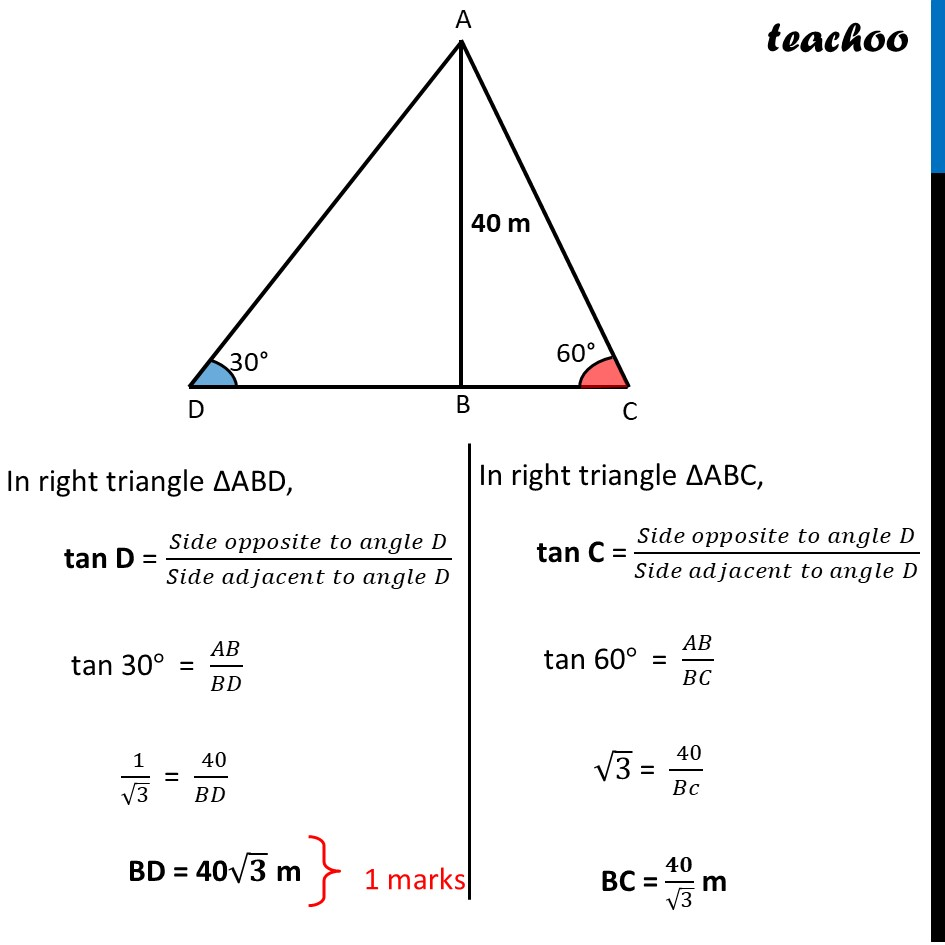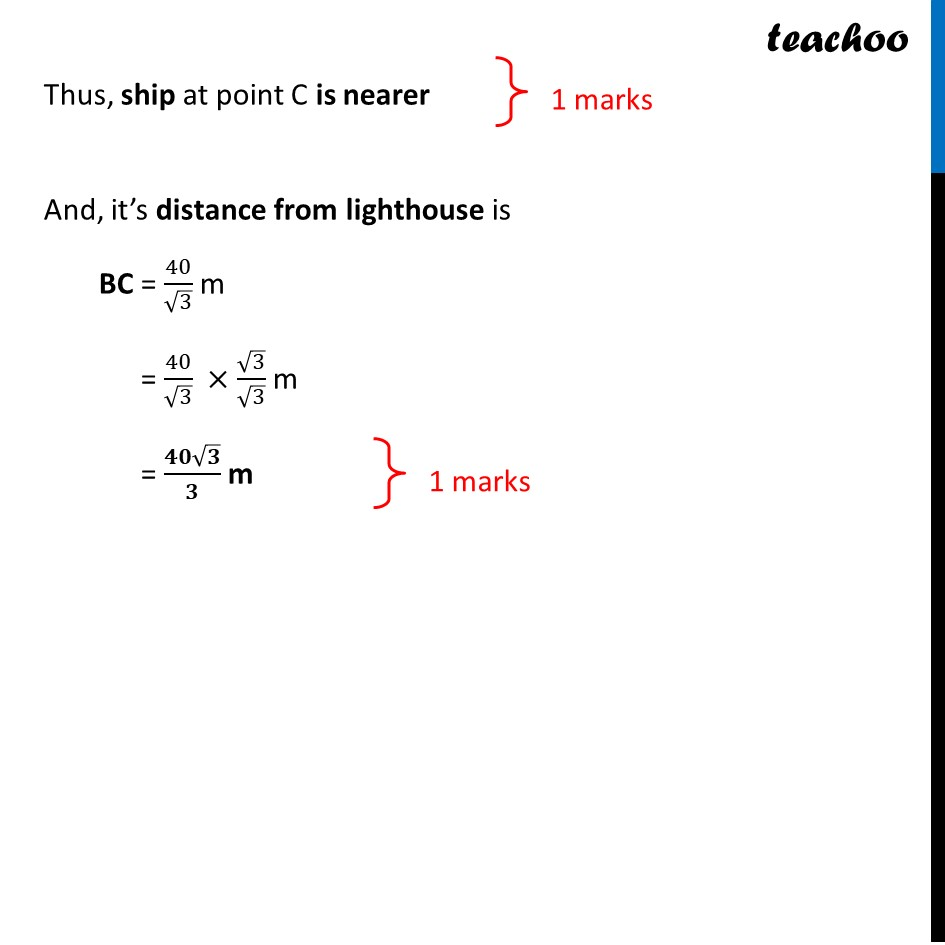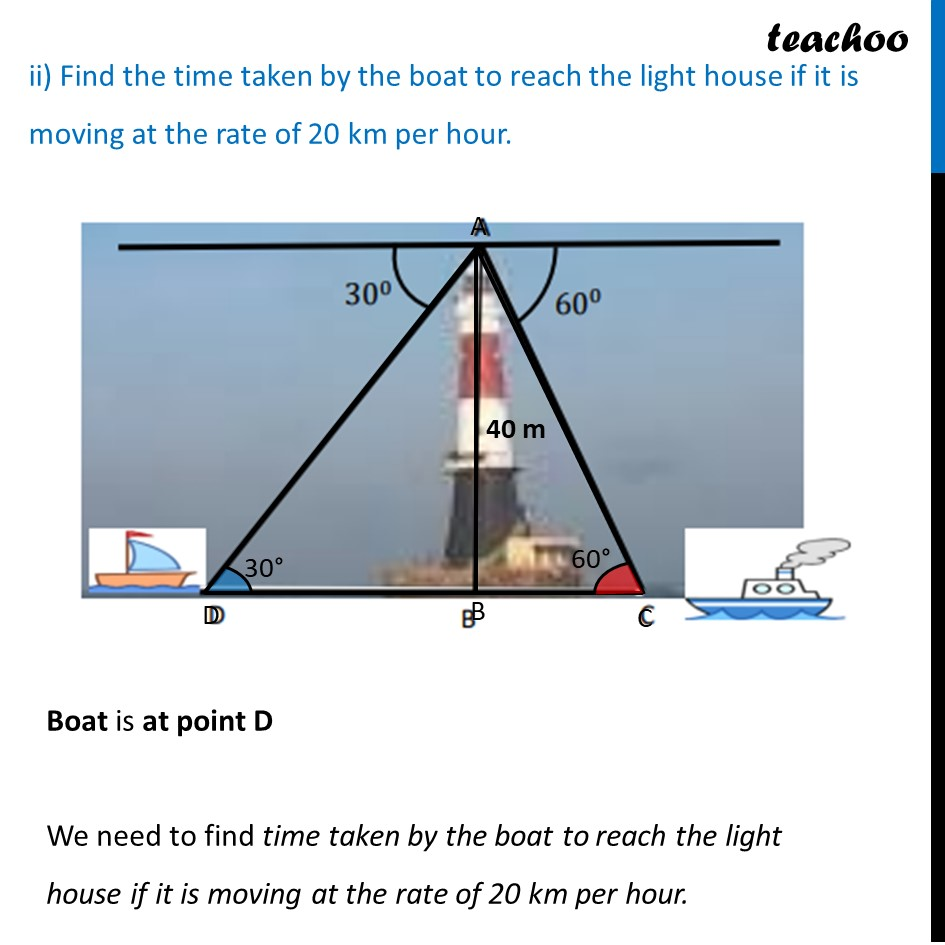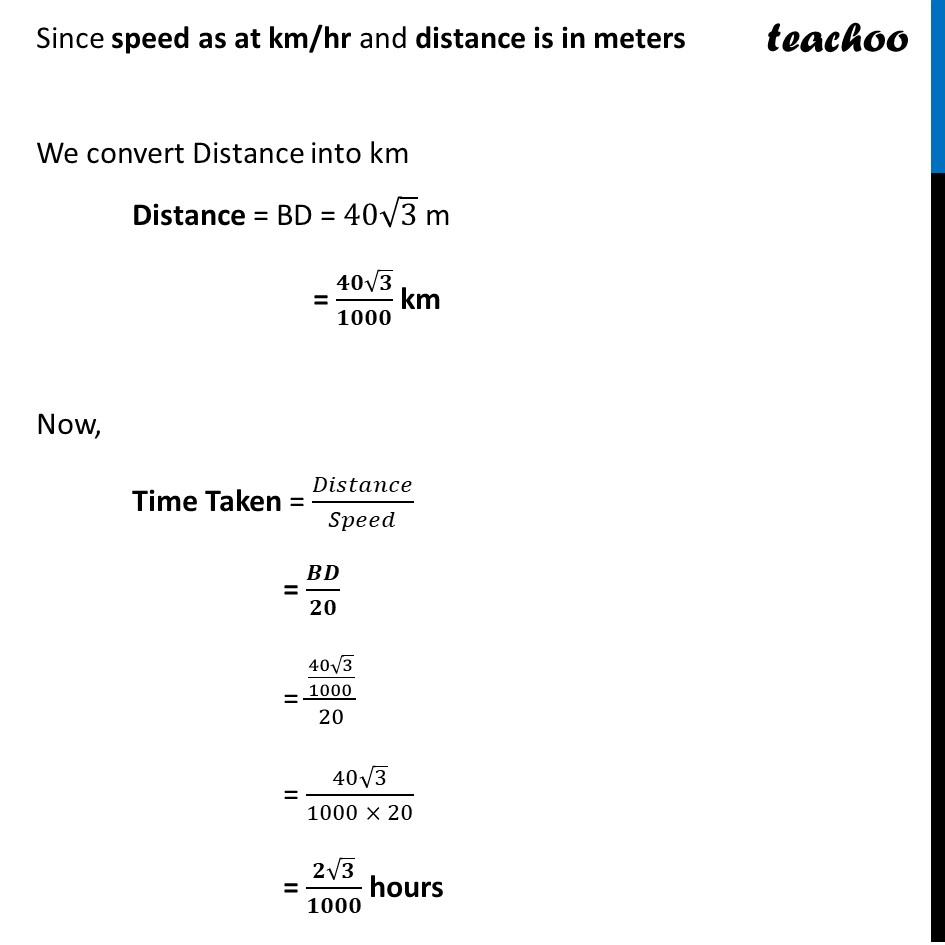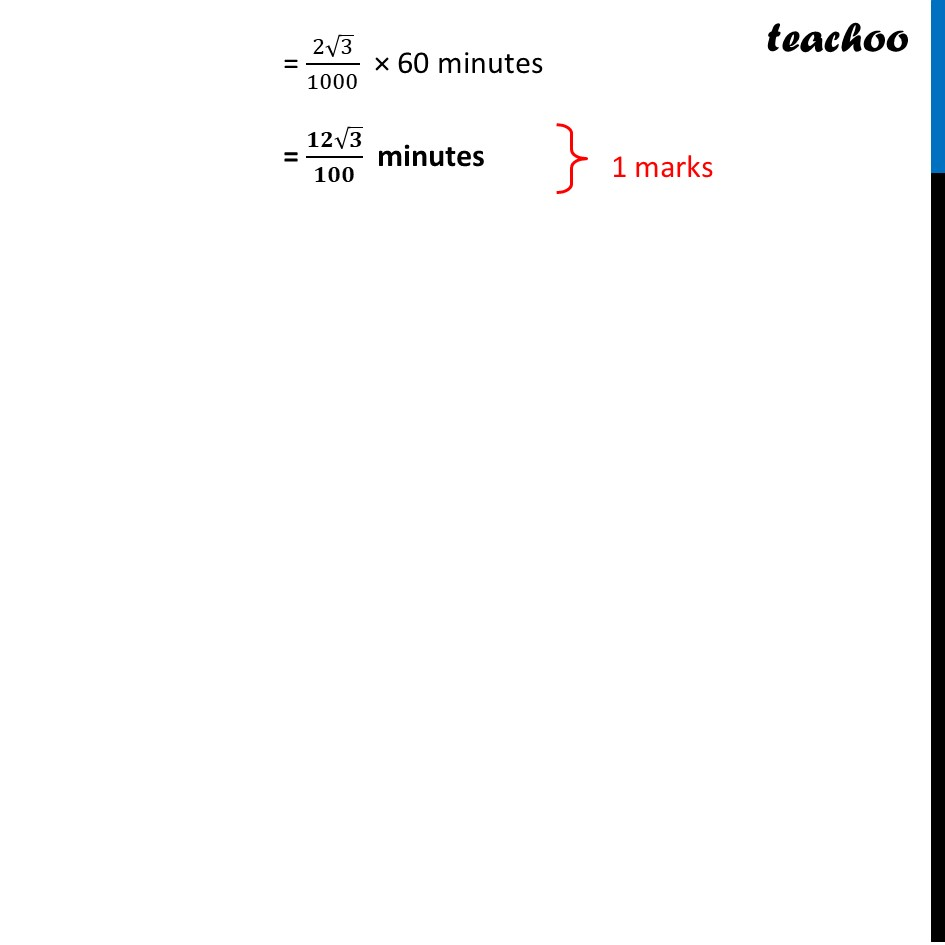Learn in your speed, with individual attention - Teachoo Maths 1-on-1 Class

### Transcript

A lighthouse is a tall tower with light near the top. These are often built on islands coasts or on cliffs. Lighthouses on water surface act as a navigational aid to the mariners and send warning to boats and ships for dangers. Initially wood, coal would be used as illuminators. Gradually it was replaced by candles, lanterns, electric lights. Nowadays they are run by machines and remote monitoring. Prongs Reef lighthouse of Mumbai was constructed in 1874-75. It is approximately 40 meters high and its beam can be seen at a distance of 30 kilometers. A ship and a boat are coming towards the lighthouse from opposite directions. Angles of depression of flash light from the lighthouse to the boat and the ship are 30° and 60° respectively. i) Which of the two, boat or the ship is nearer to the light house. Find its distance from the lighthouse? ii) Find the time taken by the boat to reach the light house if it is moving at the rate of 20 km per hour. Since Angle of depression = Angle of elevation ∴ ∠ ADB = 30° and ∠ ACB = 60° We need to find BD and BC In right triangle ΔABD, tan D = (𝑆𝑖𝑑𝑒 𝑜𝑝𝑝𝑜𝑠𝑖𝑡𝑒 𝑡𝑜 𝑎𝑛𝑔𝑙𝑒" " 𝐷)/(𝑆𝑖𝑑𝑒 𝑎𝑑𝑗𝑎𝑐𝑒𝑛𝑡 𝑡𝑜 𝑎𝑛𝑔𝑙𝑒" " 𝐷) tan 30° = 𝐴𝐵/𝐵𝐷 (" " 1)/√3 = (" " 40)/𝐵𝐷 BD = 40√𝟑 m In right triangle ΔABC, tan C = (𝑆𝑖𝑑𝑒 𝑜𝑝𝑝𝑜𝑠𝑖𝑡𝑒 𝑡𝑜 𝑎𝑛𝑔𝑙𝑒" " 𝐷)/(𝑆𝑖𝑑𝑒 𝑎𝑑𝑗𝑎𝑐𝑒𝑛𝑡 𝑡𝑜 𝑎𝑛𝑔𝑙𝑒" " 𝐷) tan 60° = 𝐴𝐵/𝐵𝐶 √3 = (" " 40)/𝐵𝑐 BC = 𝟒𝟎/√3 m Thus, ship at point C is nearer And, it’s distance from lighthouse is BC = 40/√3 m = 40/√3 ×√3/√3 m = (𝟒𝟎√𝟑)/𝟑 m ii) Find the time taken by the boat to reach the light house if it is moving at the rate of 20 km per hour. Boat is at point D We need to find time taken by the boat to reach the light house if it is moving at the rate of 20 km per hour. Since speed as at km/hr and distance is in meters We convert Distance into km Distance = BD = 40√3 m = (𝟒𝟎√𝟑)/𝟏𝟎𝟎𝟎 km Now, Time Taken = 𝐷𝑖𝑠𝑡𝑎𝑛𝑐𝑒/𝑆𝑝𝑒𝑒𝑑 = 𝑩𝑫/𝟐𝟎 = ((40√3)/1000)/20 =(40√3)/(1000 × 20) = (𝟐√𝟑)/𝟏𝟎𝟎𝟎 hours = (2√3)/1000 × 60 minutes = (𝟏𝟐√𝟑)/𝟏𝟎𝟎 minutes Since speed as at km/hr and distance is in meters We convert Distance into km Distance = BD = 40√3 m = (𝟒𝟎√𝟑)/𝟏𝟎𝟎𝟎 km Now, Time Taken = 𝐷𝑖𝑠𝑡𝑎𝑛𝑐𝑒/𝑆𝑝𝑒𝑒𝑑 = 𝑩𝑫/𝟐𝟎 = ((40√3)/1000)/20 = (40√3)/(1000 × 20) = (𝟐√𝟑)/𝟏𝟎𝟎𝟎 hours = (2√3)/1000 × 60 minutes = (𝟏𝟐√𝟑)/𝟏𝟎𝟎 minutes